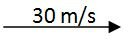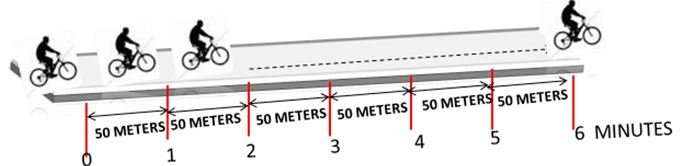The motion of a body without acceleration is known as uniform or steady velocity. Velocity is the speed of a body in particular direction.

#### Example:

A car is moving towards east with a velocity of 30m/s. The same is represented asMotion in a straight line can be represented by using "+" or "-"sign.
+30m/s (velocity of 30m/s towards east)
-30m/s (velocity of 30m/s towards west)

When the body is in uniform motion it covers equal displacement in equal interval of time.
For a body moving with steady velocity acceleration is zero.
If a body moves with uniform velocity, Initial velocity (u) and Final velocity (v) are equal.
From the definition of acceleration: Acceleration = change in velocity / time
∴ Acceleration = v – u / t = 0 / t ( v = u ) =0
Instantaneous velocity and average velocity are same for a body moving with steady velocity.

#### Worked Example:

Example 1: A person is moving on bicycle with steady velocity of 50 meters per minute. Calculate the distance travelled by the person in 4 minutes.

A. 50 Meters
B. 100 Meters
C. 200 Meters
D. 400 Meters#### Solution:

Step 1: Steady velocity of the person = 50 meters in one minute
Step 2: Distance covered by the person in one minute = 50 meters
Step 3: Distance covered by the person in 4 minutes = 4 x 50 = 200 meters

Example 2: A car starts from rest, and covers a distance of 30 meters in 5 seconds, then it moves with a steady velocity of 20m/s for next 10 seconds and then it comes to rest after 2 seconds by covering a displacement of 5 meters. Total how much distance was covered in this journey?

A. 55 meters
B. 235 meters
C. 200 meters
D. 150 meters

#### Solution

Step 1: Distance travelled by the car in 1st 5 seconds = 30 meters
Step 2: Distance travelled by the car in next 10 seconds = 10 X 20 = 200 meters (20m/s steady velocity for 10 seconds)
Step 3: Distance travelled by the car in the last 2 seconds = 5 meters
Step 4: Total distance travelled by the car = 30m + 200m + 5m = 235 meters

Example 3: A body is moving with a uniform velocity. If its instantaneous velocity at 4th second is 80m/s., what is the average velocity of the body in first 10 seconds?

A. 10m/s
B. 40m/s
C. 100m/s
D. 80m/s